## Rationalizing the Ir-rational

Pythagoras

The irrationality of the square root of 2, (that is, the number cannot be expressed in a ratio of whole numbers, it is ir-ratio-nal, not-rational), was a disturbing problem for Pythagoras, the great Greek mystic philosopher and mathematician, who, because of the numerical harmonies he had found in music-theory came to the profound insight that: ‘All is number’. This we can understand today to mean that all wave patterns and their resonances which are the basis of the phenomenal world from the tiniest quantum vibrations to the huge radio waves, are expressible in natural number ratios or rational numbers.
The square root of 2, though, because it could not be expressed in whole numbers threatened the very foundation of his most precious insight and many stories are told about how much of a taboo the whole question of square root 2 became in the Pythagorean brotherhood.
The Pythagoreans have had a lasting, and not fully appreciated, influence on European thinking, not in the least because Plato was much indebted to their ideas and banned anybody who had no idea of geometry from his Academy.

In the following I argue for a method by which it is easy to gain insight in the factors that make up a large number and the geometrical relationships that are involved.

The Second Pyramid (Khafre)
In the analysis of the Giza Pyramid proportions we will see that the Second Pyramid is based on the Pythagorean theorem of rectangular triangles of which 3 : 4 : 5, that is 3×3+4×4 equals 5×5, or in formula: a²+b²=c², is the simplest. It is though questionable whether the Egyptians had found the mathematical proof, as Pythagoras probably did; they knew the empirical proof as they did by solving the problem of the irrationality of square root 2 mathematically by big numbers and practically by creating the remen (RR) as a measure which has a sqrt(2) relation to the royal cubit (RC), which proves their awareness of the incompatibility problem on small scales.
Though Pythagoras studied in Egypt, and it is probable he got the basics of his theorem from his studies there, it is quite sure that by that time (roughly 2000 years after the building of the Giza complex) the mathematical theorem, stored in the Great & Third Pyramid design, was already long lost (it is not in the Rhind papyrus, ea), otherwise he would not have agonized about the problem of square root 2 so much, as this was beautifully solved in the proportions of these two close pyramids and is the core of the mathematical theorem presented in these pages. (99-126-140-198; 140/99=1.414..; 99/70=1.414.. and 140/126=10/9, TP)
Probably because the Second Pyramid’s Pythagorean theorem has Euclidean precision and is easier to understand, it appealed more to subsequent Pharaohs ( after Sneferu’s Giza lineage) of which several got their pyramids built according to those principles (Teti, Pepi, e.a). This may have been the reason the theorem of the Great Pyramid got lost, the more so because it was probably a divine and secret wisdom, only known to Pharaohs and their inner circles, since it understood the Earth as a sphere and measured it. No pyramid was built again on this theorem of identical square and circle circumference.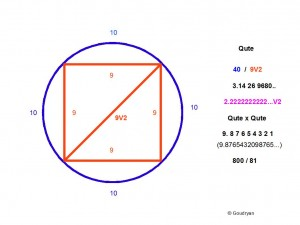We have found that any square’s perimeter inscribed in a circle in Euclidean space approximates the ratio 9 : 10, that is, in circumference (9,00342…), we can thus see what this means for our earlier model where the circumference was 22 and the diameter 7. (the two incompatible ratios 11:7 and 10:9 are the two parameters our model is based on, they are complementary though, they fit, that is why all this math works flawlessly and the numbers lead the way; I only hold the lamp to enlighten)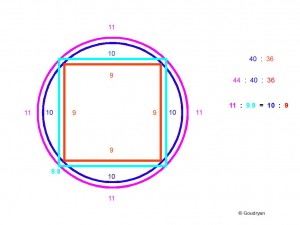We see here that just as the square side 9 fits the circle quarter arc 10, so does circle quarter arc 11 circumscribe a square with sides 9.9, the ratio remaining 10 : 9. ( Note: the dark blue number 10 relates to the dark blue circle, and 9.9 to the light blue square!, remember we deal with ratios, not with actual lengths)
Because we need whole numbers in the Stone Age we multiply by 10 and we get natural numbers again in 110:99, which is again equal to 10:9.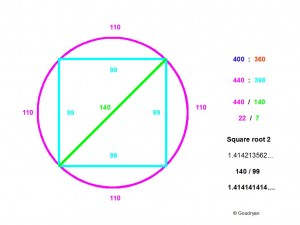We know that a circle of circumference 22 has diameter 7, this is what we start from in our natural number approximations, Pi as 22/7, so a circle of 20×22=440 has a diameter of 20×7=140, when we insert the 140 as diameter it is also the diagonal of the square with side 99, so our ‘rational square root of 2′ becomes 140/99, which is 1.414141414…. a period number and only differs from 1.4142135623730…. (which is the true irrational square root 2) by less than one ten-thousandth!
This then is the integration of the two incompatible ratios 11:7 and 10:9 by approximation and it is the theorem of the Great Pyramid, (4×440=1760 as perimeter and radius 280)  expressing the dimensions of a (hemi-)sphere, the Earth. QED

Twin values

Surely, there is a twin value for 140/99 in the ratio 99/70 or 198/140, which has the value 1.414285714…
If we compare the two values with square root 2 we get the following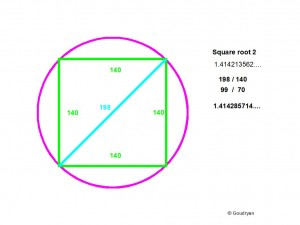140/99   = 1.414 14 14 14….
sqrt 2     = 1.414 21 35 62….
198/140 = 1.414 28 57 14….

We see that sqrt 2 lies ‘exactly’ in between our numbers and this is why in use this evens out, the best rational approximation of sqrt 2 following from our system is ((140/99)+(198/140))/2 or 19601/13860, which is 1.414213564 213564…(periodic). This is an intriguing number itself also, following from this theorem, as it is ((99×99)+(70V2x70V2)) / 2x7x9x10x11, so it holds all and only the natural numbers which form the heart of the theorem. (2, 11, 7, 9, 10) (213564=2^2 x 3 x 13 x 37^2)
The above 198 unit diameter/diagonal is the diameter of Orkney’s Ring of Brodgar in Megalithic Ell (ME), (as it is of the Third Pyramid’s side length in RC at platform level) but, conspicuously, it does not result in a whole number circumference in Megalithic Ell (622.28…..with 22/7), but since the diameter of  Brodgar can also be measured as 126 Megalithic Yards (0.8228m) this results in a circumference of 396 MYards for its circumference (4×99=396).
We will see, though, how Stone Age man solved this practical incompatibility problem (The Bone Measures) maybe hundreds of years before the Egyptian Pyramid builders, showing their deep understanding of the problem of incompatibility at small scales by forming different units, like the Egyptians. How practical they were!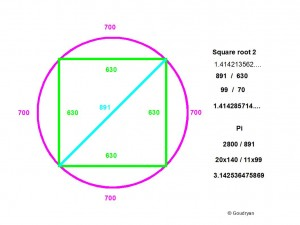The above problem can be solved by multiplying by 9 which produces another value for Pi which suits those occasions when the diagonal is not a multiple of 7 but of 11 as is the case in these two examples. This results in the other rational number for Pi: 2800/891 = 3.14 25 364758… [ (2800/891) x (22/7) = 800/81 = Pi-squared in our system]
Our diameter is then 9 x 198 = 1782, this times 2800/891 gives 5600 perimeter (40 x 140 / 18 x 99)
We can summarize our new-found values for Pi as follows:
Euclidean Pi     : 3.14 15 9265….
22/7                   : 3.14 28 57 142857 14…..      (α)
2800/891         : 3.14 25 36475869809……    (β)
((α) + (β))/2     : 3.14 26 9680  936347………. (rational Qute)
20V2/9              : 3.14 26 9680  495927…….     (Qute) (irrational)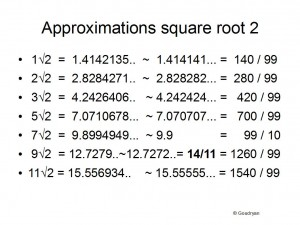The precision of this model and the reason it evens out is that all the values of the numbers 1-14, which are the only relevant numbers in the whole basic system we use, multiplied by sqrt(2), can be translated into rational numbers of which the numerator is a multiple of 140 and the denominator 99 or a full natural number, the exception is 7V2, but here an inversion takes place because 9.9 becomes 99 as denominator when 70V2 is involved.  Also  11V2   can be brought back to  14/9 = 15.5555….

All the differences with the real values are minimal (in the thousandths) and in most ordinary practical situations trivial and in terms of resonance irrelevant.

In general this means that any square root irrational number can be ‘rationalized’ by repeating the second and third or third and fourth decimal number and multiply by 99 or 990 so that a close approximate natural number will appear.

√5 = 2.236 0679…  we write  2.23232323 x 99 = 221 so that  √5 can be written as 221/99  (see factor 13 here! 13×17=221), also :
2.236 36 36 36   x 990 =  2214  so that it can be written more precise as  2214/990 = 123/55, it depends on context

√7 = 2.645 75… we write  2.64646464 x 99 = 262  so that 7 can be written as  262/99
or     2.645 45 45 45 x 990 = 2619  so that it gets more precise as  291/110

This is a key tool in the adaptation of irrational numbers to our system while the approximations never exceed the thousandths.

A beautiful example is  √3 = 1.73205080….     written as  1.732323232 x 990 = 1715  it becomes 1715/990  which can be written as  343/198 !!! This is the third power of 7 divided by  2 x 99 so it is   7³/ 22×9  a kind of acrobatic inverse of 22/7.
This also adds the beautiful relation √ 3 : √2 as  49 : 40       (343/198) : (280/198) = 343 : 280 = 49 : 40

We see here that all is perfectly restricted to the numbers of the calculus, again and again. This beautiful approximation of √3 makes it possible to incorporate the cubes in- and around spheres to our system. A cube with an axis of 343 renders a diameter of 343 for its circumscribed sphere which then has a circumference of 343 x 22/7 = 1078 ( 2 x 7 x 7 x 11). This sphere fits in a cube of 343³ = 40,353,607 which is more than 40 million units, starting with a radius of 126 units and a few transformations.

Rationalizing the irrational is a great way of getting direct insight in the approximate whole number ratios that lie behind a complex number, or a complex reality.

***********

In a further analysis we will see that Reality could not even exist if the incompatability were otherwise, but that is in the ontological questions of resonance and the ‘cosmic deep field’.